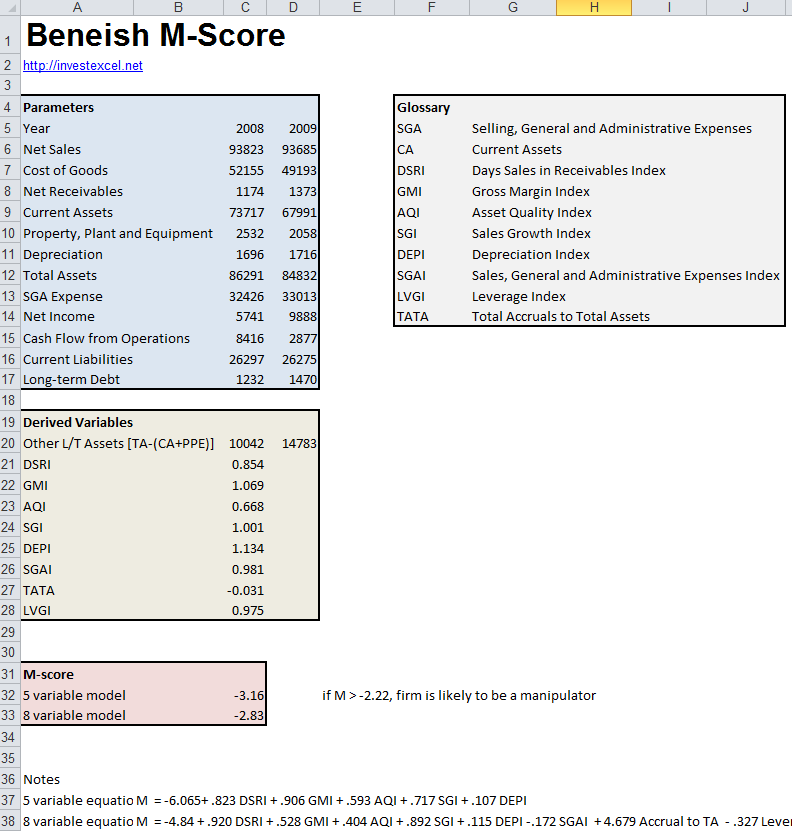# Beneish M-Score

Determine if a company has manipulated earnings with the Beneish M-Score. It’s simply a mathematical formula which uses data from a company’s financial reports.

Companies facing financial distress sometimes manipulate earnings by altering depreciation rates, delaying when expenses are recognized, registering sales early or other creating accounting tricks. This may not necessarily be illegal, but can be used to disguise if a company is suffering financial difficulty (often to maintain share price).

In 1999 Professor Messod Beneish published an empirical equation that employs eight financial ratios to detect earnings manipulation. The equation is

M = -4.84 + 0.92 DSRI + 0.528 GMI + 0.404 AQI + 0.892 SGI + 0.115 DEPI – 0.172 SGAI + 4.679 TATA – 0.327 LVGI

where

• Days Sales in Receivables Index (DSRI). This measures the increase in receivables and revenues between two reporting periods (the average for well-run companies is 1.031, while those companies that have manipulated sales have an average value of 1.465%).
• Depreciation Index (DEPI)
• Sales Growth Index (SGI)
• Leverage Index (LVGI)
• Total Accruals to Total Assets (TATA)
• Gross Margin Index (GMI)
• Asset Quality Index (AQI)
• Sales, General and Administrative Expenses Index (SGAI)
These parameters are calculated from data in company financial reports issued in two consecutive years. The following data is needed.
• Net Sales
• Cost of Goods
• Net Receivables
• Current Assets
• Property, Plant and Equipment
• Depreciation
• Total Assets
• Selling, General and Administrative Expenses,
• Net Income
• Cash Flow from Operations
• Current Liabilities
• Long-Term Debt
For example, DSRI is defined as

A latter modification to the M-Score equation eliminated some of the less significant parameters (SGAI, DEPI and LVGI), giving this five-parameter equation

M = -6.065 + 0.823 DSRI + 0.906 GMI + 0.593 AQI + 0.717 SGI + 0.107 DEP

An M-Score greater than -2.22 indicates a high probability of earnings manipulation.

Professor Beneish backtested the equation against the financial reports released by companies between 1982 to 1992 (some of which had manipulated earnings). The M-Score correctly identified 76% of those companies that had manipulated earnings. 17.5% of false-positives were returned.Beniesh later determined the effectiveness of the M Score as a stock picking tool. A strategy based on the M-Score gave a yearly hedged returned of 14%  (see “The Predictable Cost of Earnings Manipulation”, 2007).

This Excel spreadsheet implements both the 5-parameter and 8-parameter version of the M-Score. Simply enter the appropriate data from two consecutive company financial reports.### 12 thoughts on “Beneish M-Score”

1. When calculating the M-Score;
-Is it the Net amount for Property, Plant and Equipment or it is the gross amount?
-For Depreciation, I am assuming is depreciation expense and not accumulated depreciation, is this correct? do we take amortization expense into account at all?
-For Long-Term Debt, are we looking at all Long-term debt or are there any exclusions?

Many thanks,

2. I think there is a mistake in the formula. When calculating indexes you need to divided t by t-1, it seems like that in spreadsheet t-1 is divided by t.

• I think you are right, there is a bad order.

• I’ve uploaded a fixed spreadsheet. Thank you for pointing this out.

3. Some of the indexes are constructed as t divided by t-1, others as t-1 divided by t. The indexes are constructed so that higher values reflect characteristics of a manipulator. For example, manipulators have declining margins. So, GMI is margin last year (t-1) divided by margin this year (t) to create values greater than 1 for manipulators. DEPI is t-1 divided by t, as well.

4. Can you provide a reference or citation for the 5 variable model?

5. You might update the reference to the ‘Predictable cost…’ paper. That paper was shelved and replaced with, ‘Earnings manipulation and expected returns’, Financial Analysts Journal, Mar/Apr 2013, coauthored with Charles Lee and Craig Nichols

6. I want to know the basis of multiplication factors (like -4.840, 0.920, 0.528, etc,) for all the variables. How these are derived?

• they likely ran a multiple regression analysis of the form M=a+b1X1+b2X2+…+b8X8, where a=-4.840 is the intercept, and the other figures are the beta (b) coefficients.

7. @Saty,
It’s a model. They derived the constants by studying the raw data, determining a value, back testing, adjusting, testing again, etc. until they derived a formula that would deliver a number usable in comparison spreadsheets.
@Craig,
Thanks for the spreadsheet. It’s a time saver.